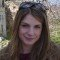Home » Java Basics » String » Substring Java ExampleKonstantina has graduated from the Department of Informatics and Telecommunications in National and Kapodistrian University of Athens (NKUA) and she is currently pursuing M.Sc studies in Advanced Information Systems at the same department. She is also working as a research associate for NKUA in the field of telecommunications. Her main interests lie in software engineering, web applications, databases and telecommunications.

# Substring Java Example

In this post, we feature a comprehensive Substring Java Example. We will show how to use Java `String substring()` API method.

## 1. Syntax

`substring()` method can be expressed in two ways:

• `String substring(int start, int end)`
• `String substring(int start)`

## 2. Parameters

• `start`

This parameter is mandatory and it specifies the starting position of the extracted string. Note that first character of string is at index 0 not 1.

• `end`

This parameter is optional and it specifies the end position of the extraction. Note that the index of end position is not included in the extraction.
If `end` parameter is missing, then the extraction starts from the starting position to the rest of the string.

## 3. Return value

The return value of `substring()` method is the extracted string.

## 4. Example

Create a java class named `SubstringExample.java` with the following code:

SubstringExample.java

```public class SubstringExample {

public static void main(String args[]) {
String str = new String("Javacodegeeks");

System.out.println("Initial string is: " + str);

System.out.println("Start position=4 and no end position specified: "
+ str.substring(4));

System.out.println("Start position=2 and end position=11: "
+ str.substring(2, 11));

// if start = end, then the extracted string will be empty
System.out.println("Start position=3 and end position=3: "
+ str.substring(3, 3).isEmpty());

// In the following cases we will get
// java.lang.StringIndexOutOfBoundException

// if (start < 0 or end < 0)
System.out.println("Start position=-2 and end position=5: "
+ str.substring(-2, 5));

// if (start > end)
System.out.println("Start position=5 and end position=2: "
+ str.substring(5, 2));
}

}```
• Output:
``````Initial string is: Javacodegeeks
Start position=4 and no end position specified: codegeeks
Start position=2 and end position=11: vacodegee
Start position=3 and end position=3: true
Exception in thread "main" java.lang.StringIndexOutOfBoundsException: String index out of range: -2
at java.lang.String.substring(Unknown Source)
at SubstringExample.main(SubstringExample.java:22)``````

As we see in the output, the `substring() `method usage is exactly what we described in the previous sections. In the first case, end parameter is omitted, so the extracted string starts from position 2 till the rest of the string.
In the second case, both parameters are defined.

In the third case, we can see that if start position is equal to the end position, then the extracted string is empty.
In the last two cases, we can see that if one of the parameters is less than 0 or start position is greater than end position, then we will have `StringIndexOutOfBoundsException.`

This was an example of Substring Java method. Download the source code of this example: JavaSubstringExample.zip(+1 rating, 1 votes)
Start the discussion Views Tweet it!

# Do you want to know how to develop your skillset to become a Java Rockstar?

## Subscribe to our newsletter to start Rocking right now!

### and many more ....